# Interpolation¶

## Heatmap (kernel density estimation)¶

Creates a density (heatmap) raster of an input point vector layer using kernel density estimation.

The density is calculated based on the number of points in a location, with larger numbers of clustered points resulting in larger values. Heatmaps allow easy identification of hotspots and clustering of points.

### Parameters¶

`Point layer` [vector: point]
Point vector layer to use for the heatmap.
`Radius` [number]

Heatmap search radius (or kernel bandwidth) in map units. The radius specifies the distance around a point at which the influence of the point will be felt. Larger values result in greater smoothing, but smaller values may show finer details and variation in point density.

Default: 100.0

`Output raster size` [number]

Allows to add the output heatmap raster layer size by specifying the rows and columns or the X and Y pixel size.

Increasing the number of rows or columns will decrease the cell size and increase the file size of the output file. At the same time, the values in `Rows` and `Columns` will also be updated, so doubling the number of rows will automatically double the number of columns and the cell sizes will also be halved. The extent of the output raster will remain the same.

`Number of rows` [number]
Total number of rows of the output raster layer.
`Number of columns` [number]
Total number of columns of the output raster layer.
`Pixel Size X` [number]
Horizontal resolution of each pixel in output raster, in layer units.
`Pixel Size Y` [number]
Vertical resolution of each pixel in output raster, in layer units.
`Radius from field` [tablefield: numeric]

Optional

Sets the search radius for each feature from an attribute field in the input layer.

`Weight from field` [tablefield: numeric]

Optional

Allows input features to be weighted by an attribute field. This can be used to increase the influence certain features have on the resultant heatmap.

`Kernel shape` [enumeration]

Controls the rate at which the influence of a point decreases as the distance from the point increases. Different kernels decay at different rates, so a triweight kernel gives features greater weight for distances closer to the point then the Epanechnikov kernel does. Consequently, triweight results in “sharper” hotspots and Epanechnikov results in “smoother” hotspots.

• 0 — Quartic
• 1 — Triangular
• 2 — Uniform
• 3 — Triweight
• 4 — Epanechnikov

Default: 0

`Decay ratio (Triangular kernels only)` [number]

Optional

Can be used with Triangular kernels to further control how heat from a feature decreases with distance from the feature.

• A value of 0 (=minimum) indicates that the heat will be concentrated in the center of the given radius and completely extinguished at the edge.
• A value of 0.5 indicates that pixels at the edge of the radius will be given half the heat as pixels at the center of the search radius.
• A value of 1 means the heat is spread evenly over the whole search radius circle. (This is equivalent to the ‘Uniform’ kernel.)
• A value greater than 1 indicates that the heat is higher towards the edge of the search radius than at the center.

Default: 0.0

`Output value scaling` [enumeration]

Allow to change the values of the output heatmap raster.

Options:

• 0 — Raw
• 1 — Scaled

Default: Raw

### Outputs¶

`Heatmap` [raster]
Raster layer with kernel density values.

### Example: Creating a Heatmap¶

For the following example, we will use the `airports` vector point layer from the QGIS sample dataset (see Downloading sample data). Another excellent QGIS tutorial on making heatmaps can be found at http://qgistutorials.com.

In Figure_Heatmap_data_processing, the airports of Alaska are shown.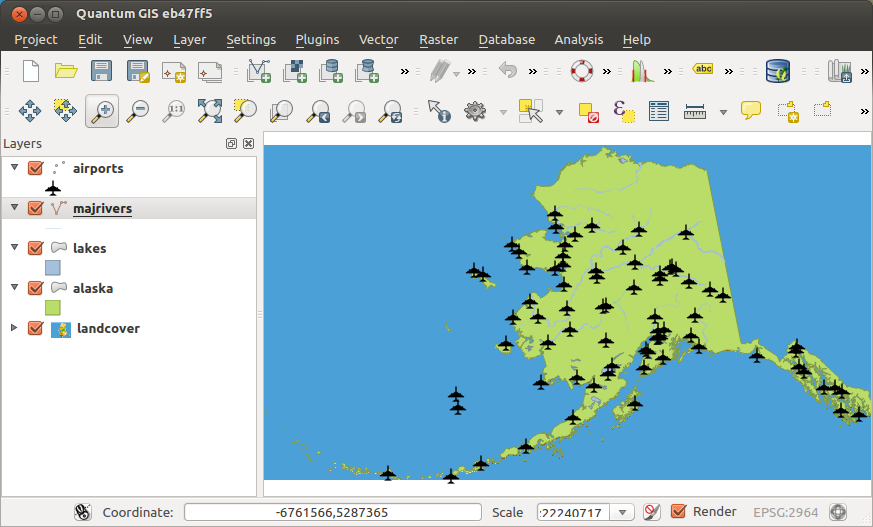1. Open the Heatmap algorithm from the Interpolation group
2. In the Point layer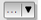field, select `airports` from the list of point layers loaded in the current project.
3. Change the Radius to `1000000` meters.
4. Change the Pixel size X to `1000`. The Pixel size Y, Rows and Columns will be automatically updated.
5. Click on Run to create and load the airports heatmap (see Figure_Heatmap_created_processing).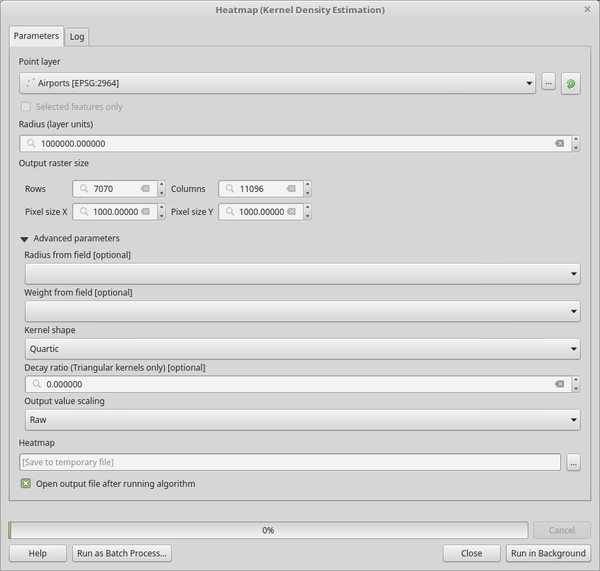The Heatmap Dialog

QGIS will generate the heatmap and add the results to your map window. By default, the heatmap is shaded in greyscale, with lighter areas showing higher concentrations of airports. The heatmap can now be styled in QGIS to improve its appearance.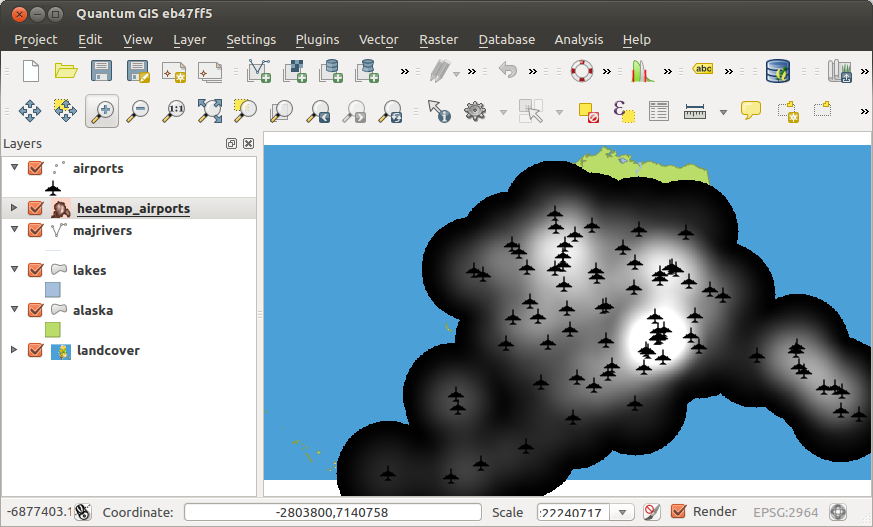1. Open the properties dialog of the `heatmap_airports` layer (select the layer `heatmap_airports`, open the context menu with the right mouse button and select Properties).
2. Select the Symbology tab.
3. Change the Render typeto ‘Singleband pseudocolor’.
4. Select a suitable Color map, for instance `YlOrRed`.
5. Click the Classify button.
6. Press OK to update the layer.

The final result is shown in Figure_Heatmap_styled_processing.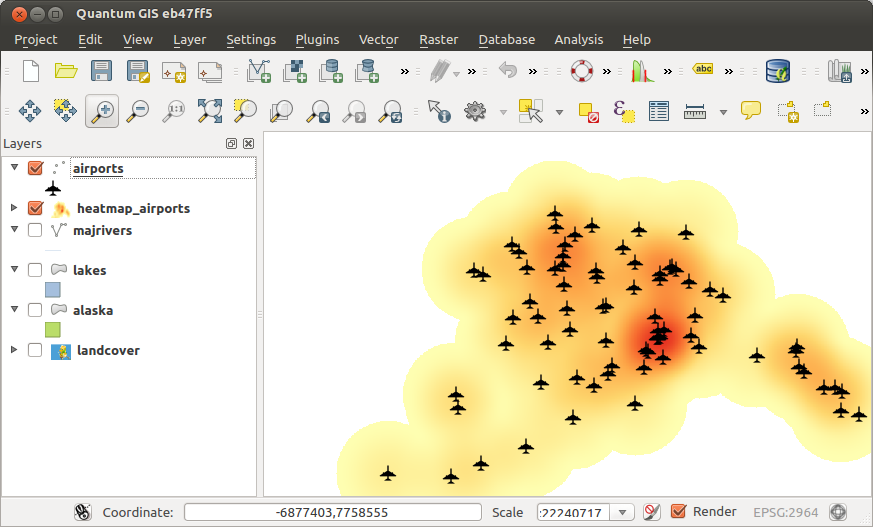Styled heatmap of airports of Alaska

## IDW Interpolation¶

Generates an Inverse Distance Weighted (IDW) interpolation of a point vector layer.

Sample points are weighted during interpolation such that the influence of one point relative to another declines with distance from the unknown point you want to create.

The IDW interpolation method also has some disadvantages: the quality of the interpolation result can decrease, if the distribution of sample data points is uneven.

Furthermore, maximum and minimum values in the interpolated surface can only occur at sample data points.

### Parameters¶

`Vector layer` [vector: point]
Point vector layer to use for the interpolation.
`Interpolation attribute` [tablefield: numeric]
Field used for the interpolation. Once you have chosen the layer and the field click on the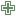button to add the layer and the field to the list. Click on the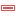button to remove the layer from the list. It is possible to insert lines or polygons as constraints for the interpolation, by specifying either `Points`, `Structure lines` or `Break lines` in the `Type` column of the table.
`Use Z-coordinate for interpolation` [boolean]
Uses the layer’s stored Z values.
`Distance coefficient Power` [number]

Sets the distance coefficient for the interpolation.

Default: 2.0

`Extent (xmin, xmax, ymin, ymax)` [extent]
Extent of the output raster layer. You have to declare the output extent by either choosing it from the map canvas, selecting it from another layer or type it manually.
`Output raster size` [number]

Allows to set the size of the interpolated output raster layer by specifying the rows and columns or the X and Y pixel size.

Increasing the number of rows or columns will decrease the cell size. Similarly, the values in `Rows` and `Columns` will be updated when one of them are changed.

`Number of rows` [number]
Total number of rows of the output raster layer.
`Number of columns` [number]
Total number of columns of the output raster layer.
`Pixel Size X` [number]
Horizontal resolution of each pixel in output raster, in layer units.
`Pixel Size Y` [number]
Vertical resolution of each pixel in output raster, in layer units.

### Outputs¶

`Interpolated` [raster]
Raster layer of interpolated values.

## TIN Interpolation¶

Generates a Triangulated Irregular Network (TIN) interpolation of a point vector layer.

With the TIN method you can create a surface formed by triangles of nearest neighbor points. To do this, circumcircles around selected sample points are created and their intersections are connected to a network of non overlapping and as compact as possible triangles. The resulting surfaces are not smooth.

The algorithm creates both the raster layer of the interpolated values and the vector line layer with the triangulation boundaries.

### Parameters¶

`Vector layer` [vector: point]
Point vector layer to use for the interpolation.
`Interpolation attribute` [tablefield: numeric]
Field used for the interpolation. Once you have chosen the layer and the field click on thebutton to add the layer and the field to the list. Click on thebutton to remove the layer from the list. It is possible to insert lines or polygons as constraints for the triangulation, by specifying either `Points`, `Structure lines` or `Break lines` in the `Type` column of the table.
`Use Z-coordinate for interpolation` [boolean]
Uses the layer’s stored Z values.
`Interpolation method` [enumeration]

There are two different choices:

• 0 — Linear
• 1 — Clough-Toucher (cubic)
`Extent (xmin, xmax, ymin, ymax)` [extent]
Extent of the output raster layer. You have to declare the output extent by either choosing it from the map canvas, selecting it from another layer or type it manually.
`Output raster size` [number]

Allows to set the size of the interpolated output raster layer by specifying the rows and columns or the X and Y pixel size.

Increasing the number of rows or columns will decrease the cell size. Similarly, the values in `Rows` and `Columns` will be updated when one of them are changed.

`Number of rows` [number]
Total number of rows of the output raster layer.
`Number of columns` [number]
Total number of columns of the output raster layer.
`Pixel Size X` [number]
Horizontal resolution of each pixel in output raster, in layer units.
`Pixel Size Y` [number]
Vertical resolution of each pixel in output raster, in layer units.

### Outputs¶

`Interpolated` [raster]
Raster layer of triangulated values.
`Triangulation` [vector: line]
Triangulation lines as vector layer.